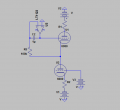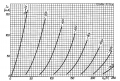# Need advice on a dual auto-bias design.

#### coinmaster

Joined Dec 24, 2015
502
So I've been working on a project for many months, or at least the concept of it with some bench experiments in between when needed.
I've pretty much gotten it all figured out thanks largely to the people on this forum.

However there is 1 small caveat that I haven't figured out yet. It's not essential to the project but it would be seriously useful if I found a solution for it.

The basic problem is thisSo let's say I have 2 vacuum tubes of the same type in push pull configuration like above.
The line between them is the output and it needs to remain at 0VDC.

So what I have here is the bottom triode being biased at a specific point on the load line, let's say V1 is -75 and V3 is -100.
This means that the grid of the bottom triode is 25v more negative than the cathode and it is operating at 75v. This is a reasonably linear area on the load line for a 6080 tube.

Tubes are not like solid state devices, they vary from one to another and drift over time. Therefore I need to use the opamp integrator "servo" on the left to bias the grid of the top triode.
The integrator samples its non-inverting input and tries to make its inverting input the same by adjusting its output which is feeding the grid of the top triode.
So effectively it will adjust the grid of the top triode until the output between the two triodes is at ground level or 0vDC.

The only time there is 0vDC between them is generally when both tubes are at an equal impedance, kind of like 2 resistors on a resistor divider.

However, what I want to do here is adjust the supply voltages to both tubes but still maintain that equal impedance.
The problem here is that while the top triode can be automatically adjusted via the integrator, the bottom triodes grid is fixed.

If I use an integrator on the bottom grid as well as the top one, the bottom one will go to 0vDC and the top one will do whatever it needs to get the output to be 0vDC, which in this scenario is to cut off conduction of the bottom tube or something equally wonky.

I tried simulating a situation where the bottom triode's grid is stabilized first before letting the bottom integrator take control but it still drops down to zero while the top integrator takes control off the offset.

I do not know why this happens exactly, I would need them both to take equal control for it to work.

I tried referencing the non-inverting input of the bottom integrator to the output of a current sense IC attached to a current source and then referencing the inverting input to another current sense IC on the cathode of the bottom triode, that way the offset mechanism wouldn't be functioning off of the output voltage but the current conduction and the servo would try to make the bottom triode conduct the current expected at the spot on the loadline that I chose for both tubes, but for some reason it didn't work either. It just cuts off the tube and for some reason both the opamp inputs were at the same potential even though the currents were not the same. Perhaps a simulated current sense IC failure?

So the only other method I can think of to have an automatic bias on both tubes is to reference the voltage difference between the top grid and the output and mirror the potential difference at the bottom triodes cathode and grid.

So for example lets say the top grid was -25v and the output was zero.
I would use some sort of mechanism that would measure a 25v difference and then keep the bottom grid at a 25v difference as well.

This way I can have the integrator keep track of the grid bias requirements at different points along the load line as I adjust the input voltages and then mirror them to the bottom.I assume this method would reach an equalibrium without wonky results but the question is what sort of equalibrium would it reach.
Assuming it did what it needed to to keep 0V at the output and kept both triodes at an equal bias setting it could easy be at any point of current along the vertical axis.

If I had the supply voltage at 100v, would it settle at a -30v grid bias or a -40v grid bias? That's a 50ma difference in current conduction.

In either case I assume I could just adjust the reference of the non-inverting input of the integrator and adjust the idle current that way.
But that still leaves me with the question of what kind of circuit I can use to sample the voltage differential of the top triodes grid and the output and then mirror that differential on the bottom triode.

Any ideas?

#### AnalogKid

Joined Aug 1, 2013
10,563
However, what I want to do here is adjust the supply voltages to both tubes but still maintain that equal impedance.
Why? Not being snotty, just asking - what is it you are trying to achieve by adjusting the two power supply voltages? It sounds like you want a second control loop to cause a certain condition based on certain inputs. What condition and what inputs? Making +/- tracking power supply outputs is simple, using a variation of the servo circuit in your schematic. Once that is in place, modulating the reference voltage for the master regulator will cause both outputs to move together. So, why do you want to do this, and what is driving the loop to a closed, stable condition?

ak

#### coinmaster

Joined Dec 24, 2015
502
what is it you are trying to achieve by adjusting the two power supply voltages?
Basically I want to manually adjust the load line operating point during operation for experiments.

It sounds like you want a second control loop to cause a certain condition based on certain inputs. What condition and what inputs?
what is driving the loop to a closed, stable condition?
Well the integrator on the top triode would be working to keep 0VDC between the two triodes and if both triodes maintain exact same voltage differential between their cathodes and grids then I would think that the conclusion is that the only way to get 0vDC between them is when they are both conducting the same amount of current.

If both tubes have are running at 75v between cathode and anode for example and both running at a 25v differential between the cathodes and grids, then based on the load line curves there are only so many places they can end up and still have 0VDC between them, meaning they should in theory end up conducting the same amount of current, I hope.

So basically lets say the top triode grid is -25v and the cathode is 0v.

This circuit I want to create would read the differential and then make sure the bottom triodes grid is also 25v more negative than the cathode.

So no matter what the top triode does the bottom triode would mirror it and the output should come to some sort of equilibrium at 0v which would assumingly be at either a point where they both have a matching impedance or they are both completely cut off.

It should be noted that I do have 350v opamps at my disposal which I will no doubt need for this.

Last edited: